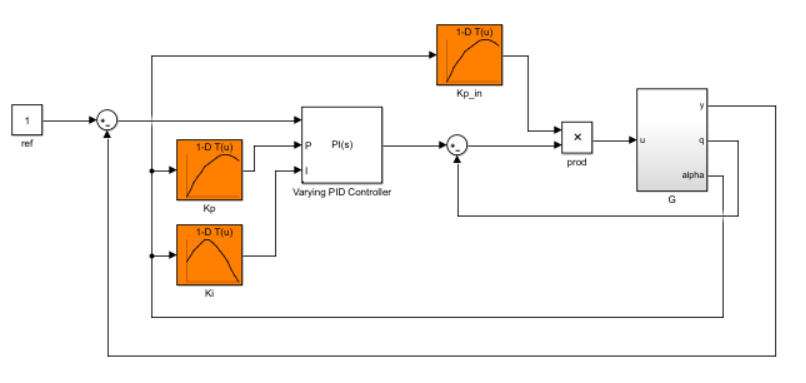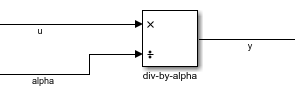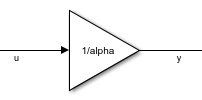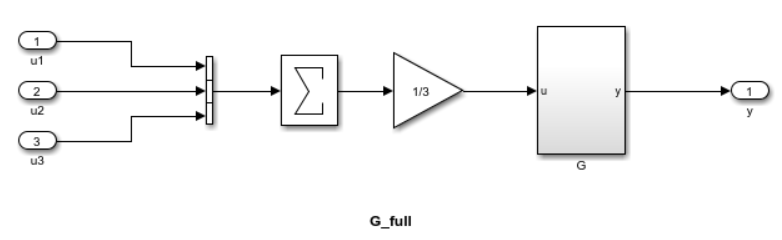## Multiple Design Points in `slTuner` Interface

For tuning a gain-scheduled control system, you must make your Simulink® model linearize to an array of LTI models corresponding to the various operating conditions that are your design points. Thus, after you obtain a family of linear plant models as described in Plant Models for Gain-Scheduled Controller Tuning, you must associate it with the `slTuner` interface to your Simulink model. To do so, you use block substitution to cause `slTuner` replace the plant subsystem of the model with the array of linear models. This process builds a family of tunable closed-loop models within the `slTuner` interface.

### Block Substitution for Plant

Suppose that you have an array of linear plant models obtained at each operating point in your design grid. In the most straightforward case, the following conditions are met:

• The linear models in the array correspond exactly to the plant subsystem in your model.

• Other than the elements you want to tune, nothing else in the model varies with the scheduling variables.

For a Simulink model `mdl` containing plant subsystem `G`, and a linear model array `Garr` that represents the plant at a grid of design points, the following commands create an `slTuner` interface:

```BlockSubs = struct('Name','mdl/G','Value',Garr); st0 = slTuner('mdl',{'Kp','Ki'},BlockSubs);```

`st0` contains a family of closed-loop linear models, each linearized at a design point, and each with the corresponding linear plant inserted for `G`. If `'Kp'`and `'Ki'` are the gain schedules you want to tune (such as lookup tables), you can parameterize them with tunable gain surfaces, as described in Parameterize Gain Schedules, and tune them.

### Multiple Block Substitutions

In other cases, the linearized array of plant models you have might not correspond exactly to the plant subsystem in your Simulink model. Or, you might need to replace other parts of the model that vary with operating condition. In such cases, more care is needed in constructing the correct block substitution. The following sections highlight several such cases.

For instance, consider the model of the following illustration.This model has an inner loop with a proportional-only gain-scheduled controller. The controller is represented by the lookup table `Kp_in` and the product block `prod`. The outer loop includes a PI controller with gain-scheduled proportional and integral coefficients represented by the lookup tables `Kp` and `Ki`. All the gain schedules depend on the same scheduling variable `alpha`.

Suppose you want to tune the inner-loop gain schedule `Kp_in` with the outer loop open. To that end, you obtain an array of linear models `G_in` from input `u` to outputs `{q,alpha}`. This model array has the wrong I/O dimensions to use as a block substitution for `G`. Therefore, you must "pad" `G_in` with an extra output dimension.

```Garr = [0; G_in]; BlockSubs1 = struct('Name','mdl/G','Value',Garr);```

In addition, you can remove all effect of the outer loop by replacing the Varying PID Controller block with a system that linearizes to zero at all operating conditions. Because this block has three inputs, replace it with a 3-input, one-output zero system.

```BlockSubs2 = struct('Name','mdl/Varying PID Controller','Value',ss([0 0 0])); ```

With those block substitutions, the following commands create an `slTuner` interface that you might use to tune the inner-loop gain schedule.

```st0 = slTuner('mdl','Kp_in'); st0.BlockSubstitutions = [BlockSubs1; BlockSubs2];```

See the example Angular Rate Control in the HL-20 Autopilot for a another case in which several elements other than the plant itself are replaced by block substitution.

### Substituting Blocks that Depend on the Scheduling Variables

Next, suppose that you have already tuned the inner-loop gain schedule, and have obtained an array `Kp_in_tuned`, of values of `Kp_in` that correspond to each design point (each value of `alpha` at which you linearized the plant). Suppose also that you have a new `Garr` that is the full plant from `u` to `{y,q,alpha}` linearized with the tuned inner loop closed. To tune the outer-loop gain schedules, you must replace the product block with the array `Kp_in_tuned`. It is important to note that you replace the injection point, the product block `prod`, rather than the lookup table `Kp_in`. Replacing the product block effectively converts it to a varying gain. Also, you must zero out the first input of the product block to remove the effect of the lookup table `Kp_in`.

```prodsub = [0 ss(Kp_in_tuned)]; BlockSubs1 = struct('Name','mdl/prod','Value',prodsub); BlockSubs2 = struct('Name','mdl/G','Value',Garr); st0 = slTuner('mdl',{'Kp','Ki'}); st0.BlockSubstitutions = [BlockSubs1; BlockSubs2];```
For another example that shows this kind of substitution for a previously-tuned lookup table, see Attitude Control in the HL-20 Autopilot - SISO Design.

The following illustration of a portion of a model highlights another scenario in which you might need to replace blocks that vary with the scheduling variable. Suppose the scheduling variable is `alpha`, and somewhere in your model, an signal `u` gets divided by `alpha`.To ensure that `slTuner` linearizes this block correctly at all values of `alpha` in the design grid, you must replace it by an array of linear models, one for each `alpha` value. This block is equivalent to sending `u` through a gain of 1/`alpha`:Therefore, you can use the following block substitution in your `slTuner` interface, where `alphagrid` is an array of `alpha` values at your design points.

```divsub = ss[(1/alphagrid), 0] BlockSubs = struct('Name','mdl/div-by-alpha','Value',divsub); st0.BlockSubstitutions = [st0.BlockSubstitutions; BlockSubs]```

Each entry in model array `divsub` divides its first input by the corresponding entry in `alphagrid`, and zeros out its second input. Thus, this substitution gives the desired result `y = u/alpha`.

### Resolving Mismatches Between a Block and its Substitution

Sometimes, the linear model array you have is not an exact replacement for the part of the model you want to replace. For example, consider the following illustration of a three-input, one-output subsystem.Suppose you have an array of linearized models `Garr` corresponding to `G`. You can configure a block substitution for the entire subsystem `G_full` by constructing a substitution model that reproduces the effect of averaging the three inputs, as follows:

```Gsub = Garr*[1/3 1/3 1/3]; BlockSubs = struct('Name','mdl/G_full','Value',Gsub); ```

Sometimes, you can resolve a mismatch in I/O dimensions by padding inputs or outputs with zeros, as shown in Multiple Block Substitutions. In still other cases, you might need to perform other model arithmetic, using commands like `series`, `feedback`, or `connect` to build a suitable replacement.

### Block Substitution for LPV Blocks

If the plant in your Simulink model is represented by an LPV System , you must still perform block substitution when creating the `slTuner` interface for tuning gain schedules. `slTuner` cannot read the linear model array directly from the LPV System block. However, you can use the linear model array specified in the block for the block substitution, if it corresponds to the design points for which you are tuning. For instance, suppose your plant is an LPV System block, `LPVPlant`, that specifies a model array `PlantArray`. You can configure a block substitution for `LPVPlant` as follows:

```BlockSubs = struct('Name','mdl/LPVPlant','Value',PlantArray); ```